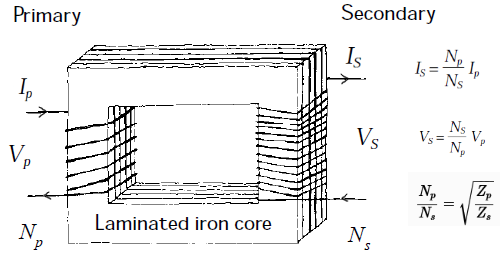# Transformer Calculator Online

Inputs:

Results:Inputs:

Results:

Inputs:

Results:

Equations Used:
$$I_s= \frac{N_p}{N_s} I_p$$, $$V_s= \frac{N_s}{N_p} V_p$$, $$N = \frac{N_p}{N_s} = \sqrt{\frac{Z_p}{Z_s}}$$

The above online Transformer calculator helps users to calculate the turn ratio from primary and secondary turns, secondary current and voltages, calculate turn ratio from primary and secondary inductance.

Some usage examples are as follows.

1. Voltage and Current in Transformer

Consider that a transformer has 400 primary turns and 2800 secondary turns. Consider that a voltage of 120V is applied at the primary then what will be the voltage at the secondary windings?

Given, Np=400, Ns=2800 and Vp=120V, Vs=?

So here,  turn ratio, $$N=\frac{N_p}{N_s}$$, and using the above online calculator we find N=0.14

We have, $$V_s=\frac{V_p}{N}$$ and so using the above calculator, Vs=840V.

Similarly, suppose that the secondary delivers 0.2A to the load then what is the current in the primary?

In this case, Is=0.2A, Ip=?

The formula to calculate the primary current is, $$I_p=\frac{I_s}{N}$$. Using the above calculator we get, Ip=1.4A.

2. Impedance Ratio

Consider we want to build a coupling transformer(impedance matching transformer) between a transistor audio amplifier and a speaker. The audio amplifier requires a load of 150Ohm for best performance while the loudspeaker has impedance of 4Ohm. What is the turn ratio for the coupling transformer?

Given, Zs = 150Ohm and Zp=4Ohm then we have, $$N = \sqrt{\frac{Z_p}{Z_s}}$$ and using the above calculator we have N=6.12. So the primary winding should have turns 6.12 times the secondary winding.

Other tutorials where the above transformer calculator is applicable are below.